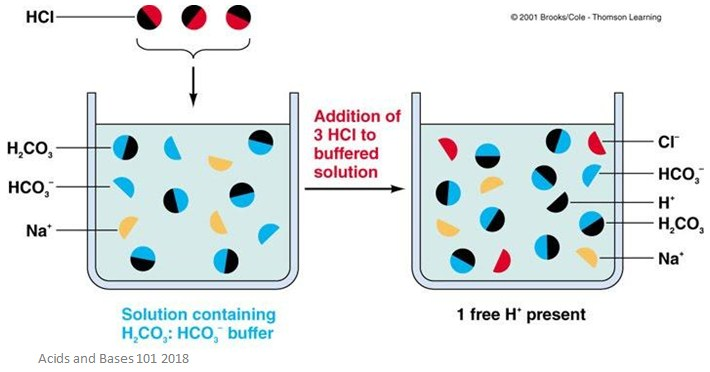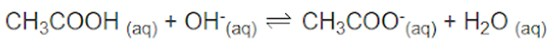Looking for revision notes that are specific to the exam board you are studying? If so, click the links below to view our condensed, easy-to-understand revision notes for each exam board, practice exam question booklets, mindmap visual aids, interactive quizzes, PowerPoint presentations and a library of past papers directly from the exam boards.

# Acid-Base Buffers

## Facts, Summary & Definition

• Acid-base buffers can resist pH changes, but not completely stop them
• They are formed of a conjugate acid-base pair
• They are important for reactions which require a specific pH range
• You can calculate the pH of a buffer solution if you know the amount of acid used, the amount of base used, and the acid dissociation constant

What are acid-base buffers?

Buffers are solutions that are able to resist pH changes – this means they are very useful when you are trying to keep the concentration of H+ ions the same. Buffers do not prevent change, they only help minimise the change in pH. They are designed to keep the concentration of hydrogen ions and hydroxide ions in a solution relatively unchanged.

Buffers are usually made of an acid-base pair – these are also called a conjugate acid-base pair. This is a buffer in which the only difference between the acid and the base is the presence of absence of a proton. For example, carbonic acid (H2CO3) has a conjugate base – the bicarbonate ion (HCO3-). As you can see, the only difference between the acid and the base is that the acid has an extra proton.

Why are buffers important?

Buffers are important for reactions or processes which need specific pH ranges to happen. This is especially useful in living systems, such as the human body, where enzymes can only work at very specific pH ranges.

For example, the bicarbonate buffer system helps maintain the pH of the blood so metabolic functions remain normal. Without this buffer system, our blood could become either very acidic or alkaline, and many of our bodily functions wouldn’t work properly. If our blood wasn’t kept at a certain pH by a buffer, lots of proteins in our body would permanently denature and they wouldn’t be able to catalyse reactions anymore.

Buffer solutions can also be used in industrial processes. They are used in everything from fabric colouring to fermentation. They can also be used to calibrate pH meters and perform chemical analysis.

A buffer can be made two ways:

1. Mixing a weak acid with one of its salts (a weak acid and its conjugate base)
2. Mixing a weak base with one of it salts (a weak base and its conjugate acid)

How do buffers work?

If there are too many H+ ions in a solution, the buffer will be able to absorb some of them and increase the pH. It works the other way too! If a solution doesn’t have enough H+ ions, the buffer can donate some, and decrease the pH of the solution.Remember that buffers cannot completely prevent pH changes – they only help to minimise pH changes.

We will use an example of a solution containing both CH3COOH and CH3COO-. Any strong base that is added to this solution will be neutralised by the acid, which removes the OH-.Any strong acid that is added to the solution will be neutralized by the acetate, which removes the H+.The amount of acid or base that a buffer can neutralize is called the buffer capacity. This is a measure of the resistance of a buffer solution to pH change when hydroxide ions are added. Put more simply, it is the amount of acid or base that can be added before the pH begins to change significantly. You can estimate the capacity of a buffer by calculating the amount of acid required to change the pH of one litre of buffer by one unit. The buffering capacity depends primarily on two things:

1. The concentration of the acid and the base in the buffer – this is because, as the concentration of acid and base components increases, the buffering capacity also increases
2. The pH of the buffer – the buffering range is usually roughly one pH unit above or below the pKa value.

Calculating the pH of a buffer solution.

To calculate the pH of a buffer solution, you need to know the amount of acid and the amount of conjugate base used. You also need to know the acid dissociation contact (Ka).

Example:  A buffer solution was made by dissolving 10.0 grams of sodium acetate in 200.0 mL of 1.00 M acetic acid.  Calculate the pH of the buffer solution.  The Ka for acetic acid is 1.7 x 10-5.

1. Start with the equation for the ionization of acetic acid and the Ka Rearrange the expression to solve for the hydronium ion concentration.

CH3COOH + H2O--> H3O+ + CH3COO-

[H3O+] = Ka[CH3COOH]

[CH3COO-]

1. Then determine the number of moles of acid and of the conjugate base.

(1.00 M CH3COOH)(200.0 mL)(1 L/1000 mL) = 0.200 mol CH3COOH

g NaCH3COO)(1 mol/82.03 g) = 0.122 mol NaCH3COO

1. Substitute these values, along with the Kavalue, into the above equation and solve for the hydronium ion concentration.  Convert the hydronium ion concentration into pH.

[H3O+] = (1.7 x 10-5)(0.200/0.122) = 2.79 x 10-5

pH = 4.56